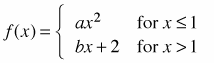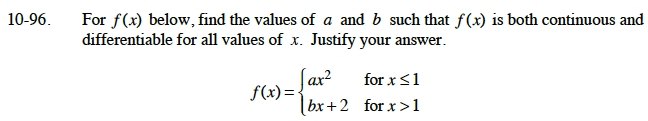### Home > CALC > Chapter 10 > Lesson 10.2.1 > Problem10-96

10-96.
1. For f(x) below, find the values of a and b such that f(x) is both continuous and differentiable for all values of x. Justify your answer. Homework Help ✎$\text{continuous: }a(1)^2=b(1)+2$

$\text{differentiable: }2a(1)=b$